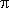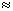# Transforming of degree measure to radian one and back

1. To find a radian measure of any angle by its given degree measure it is necessary to multiply:
a number of degrees by/ 1800.017453, a number of minutes – by/ (180 · 60 )0.000291,
a number of seconds  –  by/ (180 · 60 · 60 )0.000005 and to add the found products.

E x a m p l e .  Find a radian measure of an angle 12° 30’ with an of the fourth accuracy
decimal place.

S o l u t i o n .  Multiply  12  by/ 180 : 12 · 0.0174530.2094.
Multiply 30 by/ (180 · 60 ) : 30 · 0.0002910.0087.
Now we find:
12°30’0.2094 + 0.0087 = 0.2181 rad.

2. To find a degree measure of any angle by its given radian measure it is necessary to multiply
180° /57°.296 = 57°17’45” ( a relative error of the result will be ~ 0.0004%,
that corresponds to an absolute error ~ 5” for a round angle 360° ).

E x a m p l e .  Find a degree measure of an angle 1.4 rad. with an accuracy up to 1’.

S o l u t i o n .  We’ll find consequently:
1 rad57°17’45” ;
0.4 rad0.4 · 57°.296 = 22°.9184;
0°.9184 · 6055’.104;
0’.104 · 606”.
So,  0.4 rad22°55’6” and hence:

1 rad57°17’45”
+
0.4 rad22°55’6”
_____________________

1.4 rad80°12’51”

After rounding this result according to the required accuracy up to 1’
we have finally: 1.4 rad80°13’.